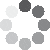Enter parameters Rectangular area
Enter parameters
LENGTH, WIDTH & DEPTH
Area length:
ft
• inches (in)
• feet (ft)
• yards (yd)
• centimeters (cm)
• meters (m)
Area width:
ft
• inches (in)
• feet (ft)
• yards (yd)
• centimeters (cm)
• meters (m)
Paver length:
in
• inches (in)
• feet (ft)
• yards (yd)
• centimeters (cm)
• meters (m)
Paver width:
in
• inches (in)
• feet (ft)
• yards (yd)
• centimeters (cm)
• meters (m)
Price per paver: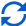Result
Area:
0
ft²
• square feet (ft²)
• square inches (in²)
• square yards (yd²)
• square miles (mi²)
• acres (ac)
• square centimeters (cm²)
• square meters ()
Number of pavers:
0
Cost:
$Enter parameters LENGTH, WIDTH & DEPTH Diameter: ft • inches (in) • feet (ft) • yards (yd) • centimeters (cm) • meters (m) Price per paver: Paver length: in • inches (in) • feet (ft) • yards (yd) • centimeters (cm) • meters (m) Paver width: in • inches (in) • feet (ft) • yards (yd) • centimeters (cm) • meters (m)Result Area: 0 ft² • square feet (ft²) • square inches (in²) • square yards (yd²) • square miles (mi²) • acres (ac) • square centimeters (cm²) • square meters () Number of pavers: 0 Cost:$(No Ratings Yet)Loading...
Similar Calculators:
Real-time graphics. Make the calculations and see the changes.
Real-time graphics. Make the calculations and see the changes.
Embed
Similar Calculators:You will agree when we say that estimating the size of your patio is not easy.

Furthermore, buying the correct number of pavers is even more difficult!

As an estimator, you want to be as accurate as possible. That’s why we built our paver calculator.

Coupled with a little preparation, it will ensure that repaving your patio goes seamlessly.

Below, we will show you just how easy the calculator is to use, how it calculates everything and how it can make you more efficient.

Contents:

## How to calculate how many pavers do I need?

• The calculator first estimates the area of the patio based on the measurements you provide.
• It then calculates how much material you will require if you provide the measurements for the individual pavers.

It estimates this using the formulae detailed below:

### Rectangular Area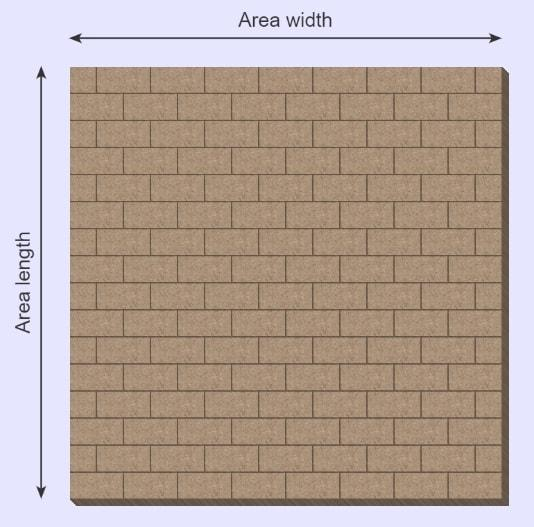The total area of a rectangular block is calculated by:

$$Patio\,Area = Patio\,Length \times Width$$

$$Paver\,Area= Paver\,Length \times Width$$

$$Number\,of\,Pavers={Patio\,Area \over Paver\,Area}$$

### Circular Area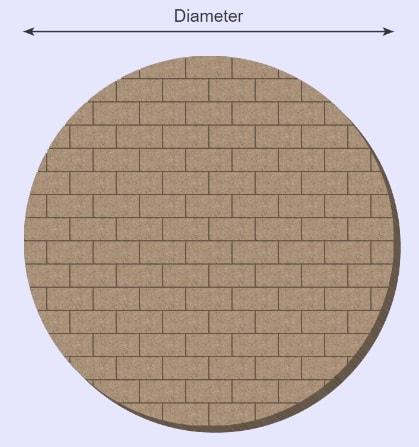The total area of a circular block is calculated by:

$$Patio\,Area= \pi\ \times \biggl({Diameter\,of\,Patio \over 2}\biggr)^2$$

$$Paver\,Area= Paver\,Length \times Width$$

$$Number\,of\,Pavers={Patio\,Area \over Paver\,Area}$$

Want to know the best part? 💰

If you have the price per paver, you can estimate the total cost for the required number of pavers using the formula:

$$Cost = Number\,of\,Pavers \times Price\,Per\,Paver$$

You can calculate the price per paver you have purchased using the formula:

$$Price\,Per\,Paver= {Total\,Cost\,of\,Paving \over Number\,of\,Pavers}$$

Confused? 🤔

See below for two examples.

Otherwise, enter your measurements and values for the concrete area and pavers in our online calculator!

## Calculation Examples

• Rectangular Concrete Block with Square Pavers

Let’s say that I have a rectangular patio with a length 1ft and width of 3ft which I want to pave with square pavers of length 0.2ft (and therefore width 0.2ft as well).

I want to know how many pavers I need and how much it will cost.

Let’s imagine that I can buy 100 stones for a cost of $20. I would enter these measurements for length and width for both the patio and the stone to work out the area. To calculate the price per paver, I would need to do the following calculation: $$Price\,Per\,Paver= {Total\,Cost\,of\,Paving \over Number\,of\,Pavers} = {20 \over 100} = 0.2$$ I would enter a cost of$0.2 in the price per paver section.

The calculator then does the following calculations:

$$Patio\,Area = Area\,Length \times Width = 1 ft \times 3 ft= 3 ft^2$$

$$Paver\,Area= Paver\,Length \times Width = 0.2 ft \times 0.2 ft = 0.04 ft^2$$

$$Number\,of\,Pavers= {Patio\,Area \over Paver\,Area} = {3 \over 0.04} = 75$$

$$Cost = Number\,of\,Pavers \times Price\,Per\,Paver = 75 \times 0.2 = 15$$

• Circular Concrete Block with Rectangular Pavers

Let’s say I have a circular patio which measures 4 feet in diameter.

I want to pave this with rectangular pavers measuring 0.2 feet in length and 0.4 feet in width.

I want to know how many pavers I need and how much it will cost.

Let’s imagine that I can purchase 20 of these bricks for a cost of $8. I would enter the measurements in the calculator for both diameter, stone length and width to work out the area. In order to calculate the price per paver I would need to do the following calculation: $$Price\,Per\,Paver= {Total\,Cost\,of\,Paving \over Number\,of\,Pavers} = {5 \over 20} = 0.4$$ I would enter a cost of$0.4 in the price per paver section.

The calculator then performs the following calculations:

$$Patio\,Area= \pi\ \times \biggl({Diameter\,of\,Patio \over 2}\biggr)^2 = \pi\ \times \biggl({4 \over 2}\biggr)^2 = 12.566 ft^2$$

$$Paver\,Area= Paver\,Length \times Width = 0.2 ft \times 0.4 ft = 0.08 ft^2$$

$$Number\,of\,Pavers= {Patio\,Area \over Paver\,Area} = {12.566 \over 0.08} = 157$$

$$Cost = Number\,of\,Pavers \times Price\,Per\,Paver = 157 \times 0.4 = 62.8$$

## But what if my measurements are in different units?

In the examples above all the measurements were in square footage.

However, in our calculator there are multiple options for the units of each measurement that are available for you to use.

You can calculate in inches, feet, yards, centimeters and meters.

Simply select the correct unit in the drop-down options and our calculator will work out the following conversion:

$$1\,foot = 12\,inches = 0.33\,yards = 30.48\,centimeters = 0.3048\,meters$$

## What if I want to figure out paving patterns?

While working with square or rectangular pavers and square or rectangular patios is straightforward, we appreciate that patterned designs can appear to be a bit more daunting.

Never fear, as long as you follow these simple rules, then working with patterned pavestones is also quite simple.

1. Assemble one repeating pattern
2. Calculate the area of that repeating pattern: $$Area\,of\,repeating\,pattern = Area\,of\,paver \times Number\,of\,pavers\,used$$
3. Calculate the number of patterns required: $$Number\,of\,patterns\,required={Area\,of\,patio \over Area\,of\,repeating\,pattern}$$
4. Calculate the number of tiles required: $$Number\,of\,tiles\,required = Number\,of\,patterns\,required \times Number\,of\,pavers\,per\,pattern$$

For example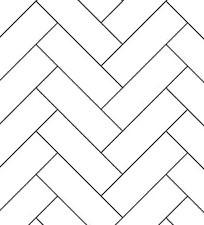Let’s say I want to use a standard Holland Herringbone pattern.

The Holland repeating pavestone is a simple V, which requires two pavers per pattern.

The area of the repeating base is therefore twice the area of a single paver.

Let’s say we have an area of 10 ft² to pave, and each stone is 0,05 ft².

Therefore, the area of the repeating pattern can be worked out by

$$2 \times 0.05 ft^2=0.1 ft^2$$

Therefore, I require 10 ft² / 0.1 ft² = 100  repeating patterns, and 200 tiles in total.

Embed this calculator on your site!Add live graphics
Copied to clipboard! Preview